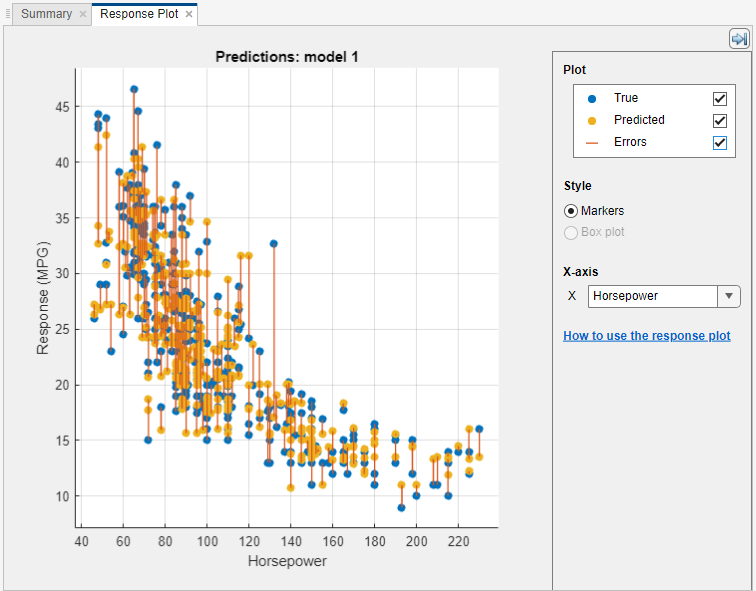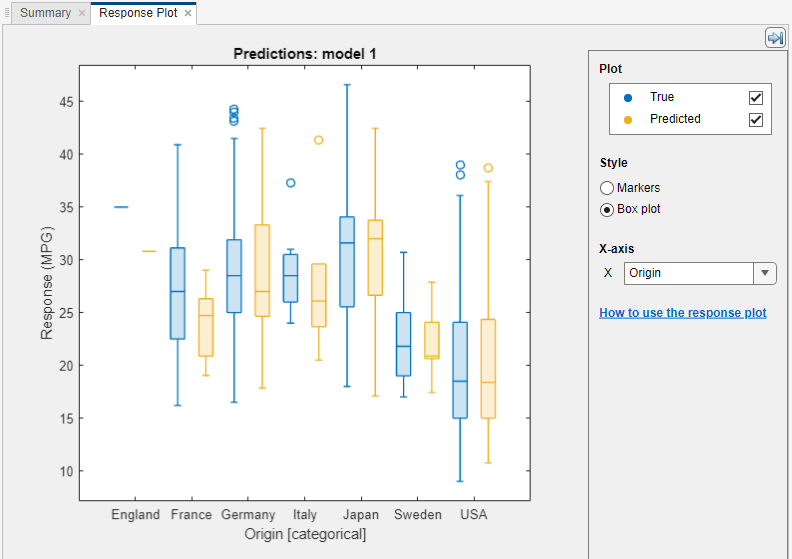## Assess Model Performance in Regression Learner

After training regression models in Regression Learner, you can compare models based on model statistics, visualize results in response plot, or by plotting actual versus predicted response, and evaluate models using the residual plot.

• If you use k-fold cross-validation, then the app computes the model statistics using the observations in the k validation folds and reports the average values. It makes predictions on the observations in the validation folds and the plots show these predictions. It also computes the residuals on the observations in the validation folds.

Note

When you import data into the app, if you accept the defaults, the app automatically uses cross-validation. To learn more, see Choose Validation Scheme.

• If you use holdout validation, the app computes the model statistics using the observations in the validation fold and makes predictions on these observations. The app uses these predictions in the plots and also computes the residuals based on the predictions.

• If you use resubstitution validation, the scores are resubstitution models statistics based on all the training data, and the predictions are resubstitution predictions.

### Check Performance in Models Pane

After training a model in Regression Learner, check the Models pane to see which model has the best overall score. The best RMSE (Validation) is highlighted in a box. This score is the root mean square error (RMSE) on the validation set. The score estimates the performance of the trained model on new data. Use the score to help you choose the best model.• For cross-validation, the score is the RMSE on all observations, counting each observation when it was in a held-out (validation) fold.

• For holdout validation, the score is the RMSE on the held-out observations.

• For resubstitution validation, the score is the resubstitution RMSE on all the training data.

The best overall score might not be the best model for your goal. Sometimes a model with slightly lower overall score is the better model for your goal. You want to avoid overfitting, and you might want to exclude some predictors where data collection is expensive or difficult.

### View and Compare Model Statistics

You can view model statistics in the Current Model Summary pane and use these statistics to assess and compare models. The Training Results statistics are calculated on the validation set. The Test Results statistics, if displayed, are calculated on an imported test set. For more information, see Evaluate Test Set Model Performance.To copy the information in the Current Model Summary pane, you can right-click into the pane and select Copy text.

Model Statistics

StatisticDescriptionTip
RMSERoot mean square error. The RMSE is always positive and its units match the units of your response.Look for smaller values of the RMSE.
R-SquaredCoefficient of determination. R-squared is always smaller than 1 and usually larger than 0. It compares the trained model with the model where the response is constant and equals the mean of the training response. If your model is worse than this constant model, then R-Squared is negative.Look for an R-Squared close to 1.
MSEMean squared error. The MSE is the square of the RMSE.Look for smaller values of the MSE.
MAEMean absolute error. The MAE is always positive and similar to the RMSE, but less sensitive to outliers.Look for smaller values of the MAE.

You can sort the models based on the different model statistics. To select a statistic for model sorting, use the Sort by list at the top of the Models pane.

You can also delete unwanted models listed in the Models pane. Select the model you want to delete and click the button in the upper right of the pane, or right-click the model and select Delete model. You cannot delete the last remaining model in the Models pane.

### Explore Data and Results in Response Plot

In the response plot, view the regression model results. After you train a regression model, the response plot displays the predicted response versus record number. If you are using holdout or cross-validation, then these predictions are the predictions on the held-out (validation) observations. In other words, each prediction is obtained using a model that was trained without using the corresponding observation. To investigate your results, use the controls on the right. You can:

• Plot predicted and/or true responses. Use the check boxes under Plot to make your selection.

• Show prediction errors, drawn as vertical lines between the predicted and true responses, by selecting the Errors check box.

• Choose the variable to plot on the x-axis under X-axis. You can choose either the record number or one of your predictor variables.• Plot the response as markers, or as a box plot under Style. You can only select Box plot when the variable on the x-axis has few unique values.

A box plot displays the typical values of the response and any possible outliers. The central mark indicates the median, and the bottom and top edges of the box are the 25th and 75th percentiles, respectively. Vertical lines, called whiskers, extend from the boxes to the most extreme data points that are not considered outliers. The outliers are plotted individually using the `'+'` symbol. For more information about box plots, see `boxplot`.To export the response plots you create in the app to figures, see Export Plots in Regression Learner App.

### Plot Predicted vs. Actual Response

Use the Predicted vs. Actual plot to check model performance. Use this plot to understand how well the regression model makes predictions for different response values. To view the Predicted vs. Actual plot after training a model, on the Regression Learner tab, in the Plots section, click Predicted vs. Actual and select Validation Data.

When you open the plot, the predicted response of your model is plotted against the actual, true response. A perfect regression model has a predicted response equal to the true response, so all the points lie on a diagonal line. The vertical distance from the line to any point is the error of the prediction for that point. A good model has small errors, and so the predictions are scattered near the line.Usually a good model has points scattered roughly symmetrically around the diagonal line. If you can see any clear patterns in the plot, it is likely that you can improve your model. Try training a different model type or making your current model type more flexible using the Advanced options in the Model Type section. If you are unable to improve your model, it is possible that you need more data, or that you are missing an important predictor.

To export the Predicted vs. Actual plots you create in the app to figures, see Export Plots in Regression Learner App.

### Evaluate Model Using Residuals Plot

Use the residuals plot to check model performance. To view the residuals plot after training a model, on the Regression Learner tab, in the Plots section, click Residuals and select Validation Data. The residuals plot displays the difference between the predicted and true responses. Choose the variable to plot on the x-axis under X-axis. Choose either the true response, predicted response, record number, or one of your predictors.Usually a good model has residuals scattered roughly symmetrically around 0. If you can see any clear patterns in the residuals, it is likely that you can improve your model. Look for these patterns:

• Residuals are not symmetrically distributed around 0.

• Residuals change significantly in size from left to right in the plot.

• Outliers occur, that is, residuals that are much larger than the rest of the residuals.

• Clear, nonlinear pattern appears in the residuals.

Try training a different model type, or making your current model type more flexible using the Advanced options in the Model Type section. If you are unable to improve your model, it is possible that you need more data, or that you are missing an important predictor.

To export the residuals plots you create in the app to figures, see Export Plots in Regression Learner App.

### Evaluate Test Set Model Performance

After training a model in Regression Learner, you can evaluate the model performance on a test set in the app. This process allows you to check whether the validation metrics provide good estimates for the model performance on new data.

1. Import a test data set into Regression Learner.

• If the test data set is in the MATLAB® workspace, then in the Testing section on the Regression Learner tab, click and select From Workspace.

• If the test data set is in a file, then in the Testing section, click and select From File. Select a file type in the list, such as a spreadsheet, text file, or comma-separated values (`.csv`) file, or select All Files to browse for other file types such as `.dat`.

In the Import Test Data dialog box, select the test data set from the Test Data Set Variable list. The test set must have the same variables as the predictors imported for training and validation.

2. Compute the test set metrics.

• To compute test metrics for a single model, select the trained model in the Models pane. On the Regression Learner tab, in the Testing section, click and select Test Selected.

• To compute test metrics for all trained models, click and select Test All in the Testing section.

The app computes the test set performance of each model trained on the full data set, including training and validation data.

3. Compare the validation metrics with the test metrics.

In the Current Model Summary pane, the app displays the validation metrics and test metrics under the Training Results section and Test Results section, respectively. You can check if the validation metrics give good estimates for the test metrics.

You can also visualize the test results using plots.

• Display a predicted vs. actual plot. In the Plots section on the Regression Learner tab, click and select Test Data.

• Display a residuals plot. In the Plots section, click and select Test Data.

For an example, see Check Model Performance Using Test Set in Regression Learner App. For an example that uses test set metrics in a hyperparameter optimization workflow, see Train Regression Model Using Hyperparameter Optimization in Regression Learner App.